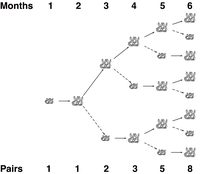# Rabbits and Recurrence Relations solved by 29519

Feb. 22, 2013, 11:50 a.m. by Rosalind Team

Topics: Combinatorics, Dynamic Programming

## Wascally WabbitsFigure 1. The growth of Fibonacci's rabbit population for the first six months.Figure 2. Erosion at Lake Mungo in New South Wales, which was initiated by European rabbits in the 19th Century. Courtesy Pierre Pouliquin.

In 1202, Leonardo of Pisa (commonly known as Fibonacci) considered a mathematical exercise regarding the reproduction of a population of rabbits. He made the following simplifying assumptions about the population:

1. The population begins in the first month with a pair of newborn rabbits.
2. Rabbits reach reproductive age after one month.
3. In any given month, every rabbit of reproductive age mates with another rabbit of reproductive age.
4. Exactly one month after two rabbits mate, they produce one male and one female rabbit.
5. Rabbits never die or stop reproducing.

Fibonacci's exercise was to calculate how many pairs of rabbits would remain in one year. We can see that in the second month, the first pair of rabbits reach reproductive age and mate. In the third month, another pair of rabbits is born, and we have two rabbit pairs; our first pair of rabbits mates again. In the fourth month, another pair of rabbits is born to the original pair, while the second pair reach maturity and mate (with three total pairs). The dynamics of the rabbit population are illustrated in Figure 1. After a year, the rabbit population boasts 144 pairs.

Although Fibonacci's assumption of the rabbits' immortality may seem a bit farfetched, his model was not unrealistic for reproduction in a predator-free environment: European rabbits were introduced to Australia in the mid 19th Century, a place with no real indigenous predators for them. Within 50 years, the rabbits had already eradicated many plant species across the continent, leading to irreversible changes in the Australian ecosystem and turning much of its grasslands into eroded, practically uninhabitable parts of the modern Outback (see Figure 2). In this problem, we will use the simple idea of counting rabbits to introduce a new computational topic, which involves building up large solutions from smaller ones.

## Problem

A sequence is an ordered collection of objects (usually numbers), which are allowed to repeat. Sequences can be finite or infinite. Two examples are the finite sequence $(\pi, -\sqrt{2}, 0, \pi)$ and the infinite sequence of odd numbers $(1, 3, 5, 7, 9, \ldots)$. We use the notation $a_n$ to represent the $n$-th term of a sequence.

A recurrence relation is a way of defining the terms of a sequence with respect to the values of previous terms. In the case of Fibonacci's rabbits from the introduction, any given month will contain the rabbits that were alive the previous month, plus any new offspring. A key observation is that the number of offspring in any month is equal to the number of rabbits that were alive two months prior. As a result, if $F_n$ represents the number of rabbit pairs alive after the $n$-th month, then we obtain the Fibonacci sequence having terms $F_n$ that are defined by the recurrence relation $F_{n} = F_{n-1} + F_{n-2}$ (with $F_1 = F_2 = 1$ to initiate the sequence). Although the sequence bears Fibonacci's name, it was known to Indian mathematicians over two millennia ago.

When finding the $n$-th term of a sequence defined by a recurrence relation, we can simply use the recurrence relation to generate terms for progressively larger values of $n$. This problem introduces us to the computational technique of dynamic programming, which successively builds up solutions by using the answers to smaller cases.

Given: Positive integers $n \leq 40$ and $k \leq 5$.

Return: The total number of rabbit pairs that will be present after $n$ months, if we begin with 1 pair and in each generation, every pair of reproduction-age rabbits produces a litter of $k$ rabbit pairs (instead of only 1 pair).

## Sample Dataset

5 3


## Sample Output

19